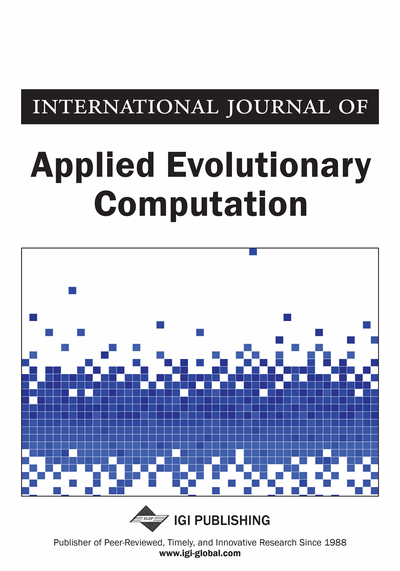# An Error Analysis Decision Making Method for Priority in Intuitionistic Preference Relation

Bhagawati Prasad Joshi (Seemant Institute of Technology, Pithoragarh, India)
DOI: 10.4018/IJAEC.2017100101
Available
\$37.50
No Current Special Offers

## Abstract

This article describes how intuitionistic fuzzy sets theory, which is the generalisation of fuzzy sets theory, is a more suitable tool for dealing with imprecise information. The information provided by the decision maker is often imprecise in many situations. So, intuitionistic preference relation is a suitable tool for such cases. The estimation of the priority vector of the intuitionistic preference relation is an important part in decision making. In this article, an intuitionistic fuzzy Choquet integral is proposed and combined with the famous error propagation formula, and developed a method for the priority of an intuitionistic preference relation to estimate the ranking of the considered alternatives. Also, an experiment has been conducted to demonstrate and implementation of the proposed approach.
Article Preview
Top

## 1. Introduction

Zadeh (1965) originally introduced the concept of fuzzy sets (FSs) and obtained attentions in many fields. Atanassov (1986) involved the hesitation degree in fuzzy sets and introduced the concept of intuitionistic fuzzy sets (IFSs), comprising by a membership function and a non-membership function. Due to the hesitation degree, IFSs theory is very useful in dealing with fuzziness under uncertain behaviour. Gau and Buehrer (1993) introduced the concept of vague sets, which is another generalization of fuzzy sets. But Bustince and Burillo (1996) clarify that the notion of vague sets is the same as that of IFSs.

Szmidt and Kacprzyk (1996) successfully used IFSs to solve group decision making problems. Szmidt and Kacprzyk (2002) introduced some solution concepts in group decision making with intuitionistic fuzzy preference relations, and gave a method based on fuzzy majority equated with a fuzzy linguistic quantifier, to aggregate the individual intuitionistic fuzzy preference relations into a social fuzzy preference. Gabriella, Yager, and Atanassov (2004) formed a generalized net model for multi-person multi-criteria decision-making process which was based on intuitionistic fuzzy graphs. An intuitionistic fuzzy interpretation of multi-person multi-criteria decision making is given by Atanassov, Pasi, and Yager (2005). Li (2005) investigated multi-attribute decision making using intuitionistic fuzzy sets and proposed several linear programming models to calculate optimal weights for criteria.

Liu and Wang (2007) proposed a series of new score functions for the multi-attribute decision making problems based on intuitionistic fuzzy point operators and evaluation function. Lin, Yuan, and Xia (2007) presented a new method for handling multi-criteria fuzzy decision-making problems based on intuitionistic fuzzy sets and linear programming model. Xu and Yager (2006) developed some geometric aggregation operators, such as the intuitionistic fuzzy weighted geometric (IFWG) operator, the intuitionistic fuzzy ordered weighted geometric (IFOWG) operator and the intuitionistic fuzzy hybrid geometric (IFHG) operator, and implemented the IFHG operator to multi-criteria decision-making problems with intuitionistic fuzzy information. Some arithmetic aggregation operators such as the intuitionistic fuzzy weighted averaging (IFWA) operator, the intuitionistic fuzzy ordered weighted averaging (IFOWA) operator and the intuitionistic fuzzy hybrid aggregation (IFHA) operator have been presented by Xu (2007a). Choquet integral has been motivated by Choquet (1954) and Denneberg (1994).

Xu and Chen (2007) developed some arithmetic aggregation operators, such as the interval-valued intuitionistic fuzzy weighted averaging (IIFWA) operator, the interval valued intuitionistic fuzzy ordered weighted averaging (IIFOWA) operator and the interval-valued intuitionistic fuzzy hybrid aggregation (IIFHA) operator, and gave an application of the IIFHA operator to multi criteria decision-making problems with interval valued intuitionistic fuzzy information. Xu (2007) developed some aggregation operators for IVIFSs, such as the interval-valued intuitionistic fuzzy weighted geometric aggregation (IIFWGA) operator and the interval-valued intuitionistic fuzzy weighted arithmetic aggregation (IIFWAA) operator, and gave an application of the IIFWGA and IIFWAA operators to multi criteria decision-making problems by using the score function and accuracy function of interval-valued intuitionistic fuzzy numbers. Xu (2007b) defined the concepts of intuitionistic preference relation, consistent intuitionistic preference relation, incomplete intuitionistic preference relation and acceptable intuitionistic preference relation, and studied their various properties.

## Complete Article List

Search this Journal:
Reset
Open Access Articles: Forthcoming
Volume 13: 4 Issues (2022): 1 Released, 3 Forthcoming
Volume 12: 4 Issues (2021)
Volume 11: 4 Issues (2020)
Volume 10: 4 Issues (2019)
Volume 9: 4 Issues (2018)
Volume 8: 4 Issues (2017)
Volume 7: 4 Issues (2016)
Volume 6: 4 Issues (2015)
Volume 5: 4 Issues (2014)
Volume 4: 4 Issues (2013)
Volume 3: 4 Issues (2012)
Volume 2: 4 Issues (2011)
Volume 1: 4 Issues (2010)
View Complete Journal Contents Listing